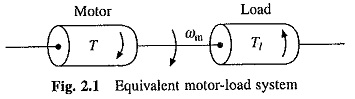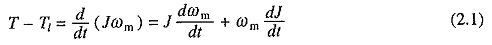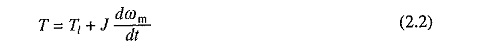## Torque Equation of Motor Load System:

A motor generally drives a load (machine) through some transmission system. While motor always rotates, the load may rotate or may undergo a translational motion. Load speed may be different from that of motor, and if the load has many parts, their speeds may be different and while some may rotate, others may go through a translational motion. It is, however, convenient to represent the Torque Equation of Motor Load System by an equivalent rotational system shown in Fig. 2.1Various notations used are:

J = Polar moment of inertia of motor-load system referred to the motor shaft, kg-m2.

ωm = Instantaneous angular velocity of motor shaft, rad/sec.

T = Instantaneous value of developed motor torque, N-m.

T1 = Instantaneous value of load (resisting) torque, referred to motor shaft, N-m.

Load torque includes friction and windage torque of motor.

Torque Equation of Motor Load System of Fig. 2.1 can be described by the following fundamental torque equation:Equation (2.1) is applicable to variable inertia drives such as mine winders, reel drives, industrial robots. For drives with constant inertia, (dJ/dt) = 0. ThereforeEquation (2.2) shows that torque developed by motor is counter balanced by a load torque T1 and a dynamic torque J(dωm/dt). Torque component J(dωm/dt) is called the dynamic torque because it is present only during the transient operations.

Drive accelerates or decelerates depending on whether T is greater or less than T1. During acceleration, motor should supply not only the load torque but an additional torque component J(dωm/dt) in order to overcome the drive inertia. In drives with large inertia, such as electric trains, motor torque must exceed the load torque by a large amount in order to get adequate acceleration. In drives requiring fast transient response, motor torque should be maintained at the highest value and Torque Equation of Motor Load System should be designed with a lowest possible inertia. Energy associated with dynamic torque J(dωm/dt) is stored in the form of kinetic energy given by (Jω2m/2). During deceleration, dynamic torque J(dωm/dt) has a negative sign. Therefore, it assists the motor developed torque T and maintains drive motion by extracting energy from stored kinetic energy.

Scroll to Top